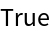Function Repository Resource:

# ApartAll

Compute a partial fraction decomposition over the algebraic closure of the rationals

Contributed by: Nikolay Murzin
 ResourceFunction["ApartAll"][expr] rewrites the rational expression expr as a sum of terms with minimal denominators in an arbitrary number field. ResourceFunction["ApartAll"][expr,var] treats all variables other that var as constants.

## Details and Options

ResourceFunction["ApartAll"][expr,"NumericFactors"True] evaluates numerically.
As with Apart, the result of ResourceFunction["ApartAll"] is often called a "partial fraction decomposition". The distinction is that Apart only factors over the rationals, whereas ResourceFunction["ApartAll"] works over the algebraic closure of the rationals.

## Examples

### Basic Examples (1)

Decompose into partial fractions:

 In:=Out=### Options (1)

#### NumericFactors (1)

"NumericFactors"True numerically evaluates factors before the computation:

 In:=Out=### Properties and Relations (2)

ApartAll works like Apart, but factorizes polynomials with :

 In:=Out=Compare outputs of Apart and ApartAll in a case where the polynomial denominator does not factor over the rationals into powers of linear polynomials:

 In:=Out=### Possible Issues (2)

Factors with multiplicity greater than one can appear in the denominator:

 In:=Out=If computation takes too long, "NumericFactors"True will do numeric factorization, which greatly speeds up the algorithm:

 In:=Out=N. Murzin

## Version History

• 1.0.0 – 24 April 2020